# SVM - Understanding the math - Duality and Lagrange multipliers

This is the Part 6 of my series of tutorials about the math behind Support Vector Machines.
Today we will learn about duality, optimization problems and Lagrange multipliers.

If you did not read the previous articles, you might want to start the serie at the beginning by reading this article: an overview of Support Vector Machine.

## Duality

In mathematical optimization theory, duality means that optimization problems may be viewed from either of two perspectives, the primal problem or the dual problem (the duality principle). The solution to the dual problem provides a lower bound to the solution of the primal (minimization) problem.  (Wikipedia)

The concept of duality is pretty simple to understand if you know what a lower bound is.

### What is a lower bound?

If you have a partially ordered set $K$ (a set having comparable elements, where the relation "less than or equal" can be used), the lower bound is an element of $K$ which is less than or equal to every element of $S$.

To be less abstract. If you pick a real number (from the partially ordered set $\mathbb{R}$) and it is less than or equal to every element of a subset of $\mathbb{R}$, then you can call this element a lower bound.

Example:

Let us consider the subset of $\mathbb{R}$:

• Because 1 is less than or equal to 2, 4 ,8 and 12, I can say that 1 is a lower bound of S.
• The same is true for -3 for instance.
• And even if it is in S we can also call 2 a lower bound of S.

Moreover, because 2 is larger than any other lower bounds, we can give it a special name, we call it the infimum (or greatest lower bound).

So in our example, you can find an infinity of lower bound, but there is only one infimum.

Note: The same logic apply with the relation "greater than or equal" and we have the concept of upper-bound and supremum.

### Coming back to duality

Now that we know what a lower bound is, what do we understand about the definition of duality? Well, this definition means that if you have a minimization problem, you can also see it as a maximization problem.  And when you find the maximum of this problem, it will be a lower bound to the solution of the minimization problem, i.e. it will always be less than or equal to the minimum of the minimization problem.

## Why do we care about duality?

It turns out that sometimes, solving the dual problem is simpler than solving the primal problem. From what we saw about lower bounds, we can see that for some problems solving the dual problem gives us the same result as solving the primal problem!   But when?

Let us look at a visual illustration.In the schema above, imagine that in our primal problem, we are trying to minimize the function at the top of the graph. Its minimum is the point $P$. If we search for a dual function, we could end up with the one at the bottom of the graph, whose maximum is the point $D$. In this case, we clearly see that $D$ is a lower bound. We defined the value $P-D$ and call it the duality gap. In this example, $P-D > 0$ and we say that weak duality holds.

In the schema below, we see that $P-D=0$, there is no duality gap, and we say that strong duality holds.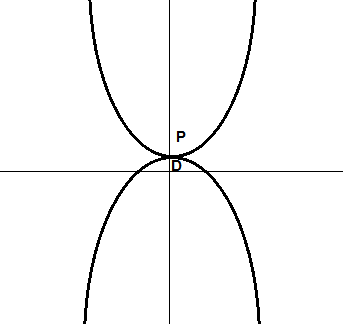## Optimization problems with constraints

#### Notation

An optimization problem is typically written:

This notation is called the standard form. You should know that there are others notations as well.

In this notation, $f$ is called the objective function (it is also sometimes called the cost function). By changing $x$ (the optimization variable) we wish to find a value $x^*$ for which $f$ is at its minimum.

There is also $p$ functions $g_i$ which define equality constraints and $m$ functions  $h_i$ which define inequality constraints.

The value we find MUST respect these constraints!

### What does it mean to respect the constraints?

Imagine you try to solve the following optimization problem:

There is no constraint, so finding the minimum is easy, the function $x^2$ is 0 when $x = 0$. This is shown with a red star on the graph below:When there is no constraint the minimum is zero

#### Equality constraints

However, what if we try to add an equality constraint? For instance, we want to find the minimum, but we must ensure that $x=1$. It means we need to solve this optimization problem:

This time, when we try $x=0$ we see that the function returns its minimum, however, we cannot say this is the solution of our optimization problem. Indeed, the constraint $x=1$ is violated. In this example, our only choice is to use $x=1$ and this is the solution.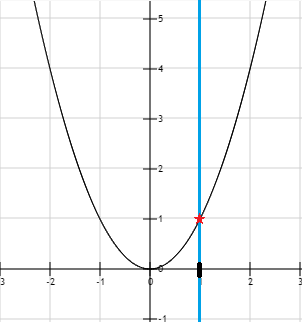With an equality constraint x=1, the minimum is 1

Looking at this example, you might feel like equality constraints are useless. This is not the case because most of the time optimization problems are performed in more than one dimension. So you could try to minimize a function $f(x,y)$ with only an equality constraint on $x$ for instance.

#### Inequality constraint

What if we now use an inequality constraint? It gives us a problem of the form:

This time, we can try more value of $x$. For instance, $x=2$ respects the constraint, so it could potentially be a solution to the problem. In the end, we find that the function $f$ has once again its minimum at $x=1$ under the constraint.Inequality constraint

On the graph above, the feasible region is shown in black bold it is a set of values of $x$ we are authorized to use. It is also called, the feasible set.

In mathematical notation we can write it:

$R$ is the set of values of $x$ for which the constraints are satisfied.

#### Combining constraints

It is possible to add several constraints to an optimization problem. Here is an example with two inequality constraints and its visual representation:Combining constraints restrict the feasible region

Note that we can also mix equality and inequality constraints together. The only restriction is that if we use contradictory constraints, we can end up with a problem which does not have a feasible set:

Indeed, for a constraint to be respected, it must be true. When there is several constraints, they all must be true.  In the example above, it is impossible for $x$ to equal 1 and to be less than zero at the same time.

For a function $f:\mathcal{X}\to\mathcal{Y}$ with one equality constraint $g(x)$ and one inequality constraint $h(x)$ the feasible set is :

### What is the solution of an optimization problem?

The optimal value $x^*$ of the optimization problem:

is:

Basically, it says in mathematical notation that the optimal value is the infimum of $f(x)$ with all the constraints respected.

## How do we find the solution to an optimization problem with constraints?

We will be using the Lagrange Multipliers. It is a method invented by the Italian mathematician, Joseph-Louis Lagrange around 1806.Joseph Louis Lagrange

### Lagrange multipliers

As often, we can find a pretty clear definition on Wikipedia:

In mathematical optimization, the method of Lagrange multipliers is a strategy for finding the local maxima and minima of a function subject to equality constraints. (Wikipedia)

The critical thing to note from this definition is that the method of Lagrange multipliers only works with equality constraints. So we can use it to solve some optimization problems: those having one or several equality constraints.

But don't worry, the Lagrange multipliers will be the basis used for solving problems with inequality constraints as well, so it is worth understanding this simpler case 🙂

Before explaining what the idea behind Lagrange multipliers is, let us refresh our memory about contour lines.

### Contour lines

It is important to understand contour lines to appreciate better Lagrange multipliers.

A contour plot is a way to visualize a three-dimensional function into two dimensions.

For instance, the picture below shows the function $x+y$ displayed in 3D (left) and with a contour plot (right):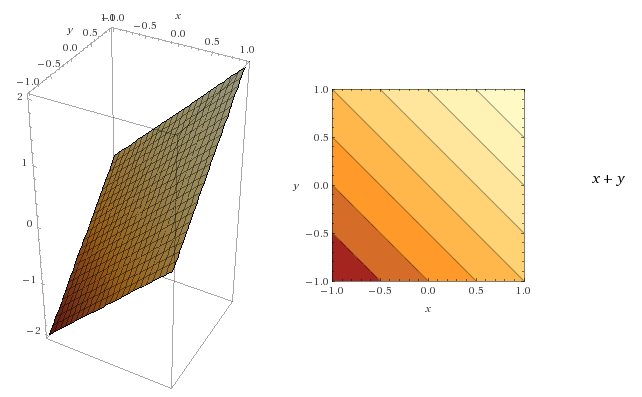Key concepts regarding contour lines:

• for each point on a line, the function returns the same value
• the darker the area is, the smallest the value of the function is

You can see the last point illustrated in the two figures below. Even if they have the same lines, the change of color allows us to draw a 3D picture of the function in our mind.Moreover, the gradient of a function can be visualized as a vector field, with the arrow pointing in the direction where the function is increasing:The gradient of the function is projected as a vector field (Wikipedia)

It turns out we can easily draw gradient vectors on a contour plot:

• they are perpendicular to a contour line
• they should point in the direction where the function is increasing

Here is a representation on two contours plots:### Back to Lagrangian multipliers

Let us consider the following optimization problem:

The objective function $f(x,y)$ and the constraint function $g(x,y)$ can be visualized as contour in the figure below: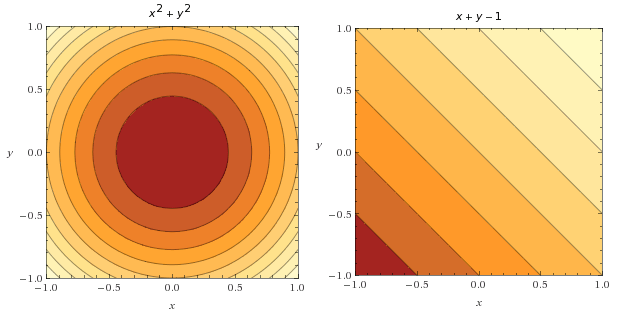It is interesting to note that we can combine both contour plot to visualize how the two functions behave on one plot. Below you can see the constraint function depicted by blue lines.
Also, I draw some gradient vectors of the objective function (in black) and some gradient vectors of the constraint function (in white).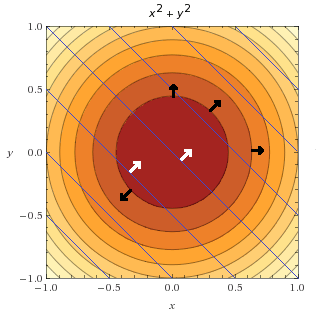However, we are not interested in the whole constraint function. We are only interested in points where the constraint is satisfied, i.e. $g(x,y)=0$.

It means that we want points where:

$x+y-1=0$

$x+y=1$

$y=1-x$

In the graph below we plot the line $y=1-x$ on top of the objective function. We saw earlier that this line is also called the feasible set.What did Lagrange find? He found that the minimum of $f(x,y)$ under the constraint $g(x,y)=0$ is obtained when their gradients point in the same direction. Let us look at how we can find a similar conclusion.

In the figure below, we can see the point where the objective function and the feasible set are tangent. I also added some objective function gradient vectors in black and some constraint gradient vectors in white.We can see that the is only one point where two vectors point in the same direction: it is the minimum of the objective function, under the constraint.

Imagine you put your finger on the first point with arrows at the top of the figure. Here you evaluate the objective function, and you find out it returns some value $v$. Now, you wish to know if there is a smaller value. You must stay on the blue line, or the constraint will be violated. So you can either go to the left or the right.

You would like to go left, but then you see that the gradient of the objective function is pointing towards the left (remember it is always pointing towards greater values) so it might not be a good idea.  To be sure, you try and go to the left. You find out that the objective function returns $v+1$, so it is increasing which is not okay. Going to the right, this time, the objective function returns $v-1$, it is good you are going in the correct direction. So you continue like that until you reach the center point where the two arrows are parallel. But then, once you move a little bit you find out that the objective function is increasing again. As you can not move right or left anymore without increasing the objective function, you conclude this point is the minimum.

#### How does it translate mathematically?

Lagrange told us that to find the minimum of a constrained function, we need to look or points were $\nabla f(x,y) = \lambda \nabla g(x,y)$.

But what is $\lambda$ and where does it come from?

It is what we call a Lagrange multiplier. Indeed, even if the two gradient vectors point in the same direction, they might not have the same length, so there must be some factor $\lambda$ allowing to transform one in the other.

Note that this formula does not require the gradients to have the same direction (multiplying by a negative number will change the direction), but only to be parallel. It is because it can be used to find both maximum and minimum at the same time (see Example 1 of this article if you want to see how).

#### How do we find points for which $\nabla f(x,y) = \lambda \nabla g(x,y)$ ?

Note that,  $\nabla f(x,y) = \lambda \nabla g(x,y)$ is equivalent to:

$\nabla f(x,y) -\lambda \nabla g(x,y) = 0$

To make things a little easier, we notice that if we define a function:

$L(x,y,\lambda) = f(x,y)-\lambda g(x,y)$ then its gradient is:

$\nabla L(x,y,\lambda) = \nabla f(x,y)-\lambda \nabla g(x,y)$

This function $L$ is called the Lagrangian, and solving for the gradient of the Lagrangian (solving $\nabla L(x,y,\lambda) = 0$) means finding the points where the gradient of $f$ and $g$ are parallels.

### Let us solve this example using the Lagrange multiplier method!

Remember, the problem we wish to solve is:

#### Step 1: We introduce the Lagrangian function

$L(x,y,\lambda) = f(x,y)-\lambda g(x,y)$

and its gradient is :

$\nabla L(x,y,\lambda) = \nabla f(x,y)-\lambda \nabla g(x,y)$

#### Step 2: We solve for its gradient

We solve :

$\nabla L(x,y,\lambda) = 0$

which means solving the following system of equations:

$\begin{cases} \frac{\partial{L}}{\partial x} = 0\\ \frac{\partial{L}}{\partial y} = 0 \\ \frac{\partial{L}}{\partial \lambda} = 0 \end{cases}$

$\begin{cases} 2x-\lambda = 0\\ 2y-\lambda = 0 \\ -x-y+1= 0 \end{cases}$

By multiplying the second equation by -1 and adding the first and second equation we get:
$2x-2y=0$
so:
$x=y$

We replace $y$ by $x$ in the third equation:
$-x-x+1=0$
$-2x+1=0$
$-2x=-1$
$x=\frac{1}{2}$

We replace $x$ by $\frac{1}{2}$ in the first equation:
$2\frac{1}{2}-\lambda=0$
$1-\lambda=0$
$\lambda=1$

We have found a solution : $x=\frac{1}{2}$$y=\frac{1}{2}$ and $\lambda=1$

We conclude that the minimum of $f$ under the contraint $g(x,y)=0$ is obtained for $x=\frac{1}{2}$$y=\frac{1}{2}$.

Let us verify if it makes sense with our graphical analysis on the graph below:The minimum of f is at x=1/2 and y=1/2

We can see that indeed the point $(x,y)=(\frac{1}{2},\frac{1}{2})$ is on the feasible set (the blue line) and it is the one we identified earlier.

## Conclusion

In this article, we learned an important concept in optimization: duality. Moreover, we discovered that an optimization problem can have equality and inequality constraints. Eventually, we learned what Lagrange multipliers are and how we can use them to solve an optimization problem with one equality constraint.

If you wish to learn more about Lagrange multipliers in the context of SVM, you can read this very good paper showing how to use them with more equality constraints and with inequality constraints.

### When will next part be ready?

There is no next part, but instead I wrote a free e-book about Support Vector Machines. That's your next step if you wish to learn more about SVM!

# SVM - Understanding the math - Convex functions

This is the Part 5 of my series of tutorials about the math behind Support Vector Machines.
Today we are going to study convex functions.

If you did not read the previous articles, you might want to start the serie at the beginning by reading this article: an overview of Support Vector Machine.

### How can we find a global minimum?

There is one simple way to find the global minimum:

1. Find all the local minima
2. Take the smallest one; it is the global minimum.

Another approach is to study the function we are trying to minimize. If this function is convex, then we are sure its local minimum is a global minimum.

Theorem: A local minimum of a convex function is a global minimum (Proof page 9)

## Convex functions

What is a convex function?

A function is convex if you can trace a line between two of its points without crossing the function line.A convex function

However, if you cross the function line, then the function is non-convex.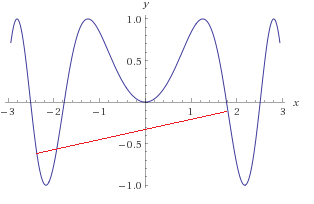A non-convex function

As you can see in the figure above, the red line crosses the function, which means it is non-convex. Note, however, that the function is convex on some intervals, for instance on [-1,+1].

You can read about a more rigorous definition of a convex function here.  But from now what is important to understand is that it is easy to find the global minimum of a convex function.

As often, there is also an "opposite" concept:  a function $f$ is concave if $-f$ is convex.A concave function

The problem here is that my original definition of a convex function is also true, I can trace a line between two points of the function without crossing the line...

So the mathematicians have been a little bit more specific, and they say that:

A function is convex if its epigraph (the set of points on or above the graph of the function) is a convex set.

But what is a convex set?

In Euclidean space, a convex set is the region such that, for every pair of points within the region, every point on the straight line segment that joins the pair of points is also within the region. (Wikipedia)

We use the same logic as before. A set of points is convex if when we pick two points belonging to the set and we trace a line between them then the line is inside the set.Which set is convex and which set is not convex?

If you guessed right, the circle and the triangles are convex sets. In the figure below I traced a red line between two points. As you can see, the line joining two points of the star leave the figure indicating that it is not a convex set.The star is not a convex set

We can now use this knowledge to determine if a function is convex.Step 1: We have a function and we wish to know if it is convex
Step 2: We take its epigraph (think of it as filling it with water but the water cannot overflow so it adds up vertically when it reaches the limits of the function)
Step 3:  If the shape of the epigraph is convex, then it is a convex function!

### How do we know if a function is convex?

The definition with the epigraph is simple to understand, but with functions with several variables it is kind of hard to visualize.  So we need to study the function:

More generally, a continuous, twice differentiable function of several variables is convex on a convex set if and only if its Hessian matrix is positive semidefinite on the interior of the convex set. (Wikipedia)

If we want to check if a function is convex, one easy way is to use our old friend the Hessian matrix. However, instead of checking if it is positive definite as we did in the previous article, this time, we need to check if it is positive semidefinite.

What is the difference?

Theorem:
The following statements are equivalent:

• The symmetric matrix $A$ is positive semidefinite.
• All eigenvalues of $A$ are non-negative.
• All the principal minors of $A$ are nonnegative.
• There exists $B$ such that $A = B^\intercal B$
(Source)

As before we will use the minors. The difference here is that we need to check all the principal minors, not only the leading principal minors. Moreover, they need to be nonnegative. (A number is positive if it is greater than zero. A number is non-negative if it is greater than or equal to zero).

#### Example: is the banana function convex?

We saw that the Hessian of our banana function was:

Its principal minors of rang 1 are:

$M_{11}$  is $200$ (we removed line 1 and column 1).

$M_{22}$  is $1200x^2-400y+2$ (we removed line 2 and column 2).

If the function is convex, these minors should be nonnegative on the interior of the convex set.  Which convex set? By definition, the domain of a convex function is a convex set. In our case when we say that a function is convex on a convex set, we are talking about its domain.
The restriction "on the interior" tells us that we should not pick points which are on the border of the set.

In our example, the function is defined in $\mathbb{R}^2$ which is a convex set. So we would need to prove that for any point we pick the principal minors are nonnegative.

We see that that minor $M_{11}$ is always positive. However, we can easily find a point for which $M_{22}$ is negative. For instance for the point $(1,4)$  $M_{22}=-399$.

As a result, we can tell the banana function is not convex.

It turns out there are several ways to prove that a function is convex. For more guidelines on the subject, refer to this paper, part 2.1.

## Why are convex functions so cool?

First, we saw that the local minimum of a convex function is a global minimum. It is a pretty good result to help us find a solution more quickly.

Moreover, in general, convex optimization problems are easier to solve. Why?

To get a better idea let us look at some figures.A convex surface

Imagine that solving the optimization problem is like throwing a marble onto a surface. In the case of the convex surface, like the one in the figure above, no matter where you put the marble, it will go directly to the center of the bowl which is the minimum of the function.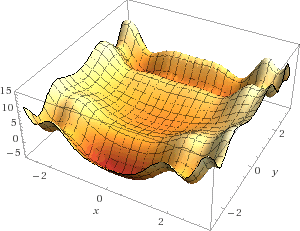A nonconvex surface

What if the surface is non-convex? Well as you can see throwing a marble randomly onto the surface has very few chances of hitting the global minimum. Instead, it is likely that the marble will fall into one of the many local minima. And when this is the case, what do you do? Do you try to push the marble to get somewhere else?  As you can see, the problem is much more complicated.

The marble analogy is interesting because it is basically what does an optimization algorithm called gradient descent.  Another way to solve an optimization problem is to use the well-known Newton's method. I encourage the interested reader to study these methods in detail and even to try implementing them.

## Conclusion

In this part, we learned what a convex set is and how to tell if a function is convex. Moreover, we saw a visual representation showing us why convex optimization is usually much simpler than non-convex optimization: because there are no local minima.

Convexity is an important concept to understand when studying optimization. Now that we know it better, we will see another important aspect called "duality". Eventually, we will see how we can solve more difficult optimization problems. Go read the Part 6 of this tutorial series to find out! Thanks for reading.

# SVM - Understanding the math - Unconstrained minimizationThis is the Part 4 of my series of tutorials about the math behind Support Vector Machines.
Today we are going to learn how to solve an unconstrained minimization problem.

If you did not read the previous articles, you might want to start the serie at the beginning by reading this article: an overview of Support Vector Machine.

It took me a while to write this article because the subject is vast and assume a lot of prior knowledge. What should I explain and what should I skip was kind of a hard line to trace. After a while, I ended up with a large Part 4 which was too long to read. So I decided to split it. Welcome, Part 4, Part 5 and Part 6!

In this article try to make it as simple as possible for everybody. However, I cannot explain everything. I will assume that you know what derivatives and partial derivatives are. You are also expected to know what a matrix, the transpose of a matrix are and how to compute the determinant of a matrix.

During the last few months, I received a lot of comments and encouragements and several hundred people subscribed to be notified when this part is published. I wish to thank all of you, and I hope you will enjoy reading it.

## Where we left.

In Part 3, we discovered that to maximize the margin we need to minimize the norm of $\textbf{w}$.

It means we need to solve the following optimization problem:

Minimize in ($\textbf{w}, b$)

$\|\textbf{w}\|$

subject to $y_i(\mathbf{w}\cdot\mathbf{x_i} + b) \geq 1$

(for any $i = 1, \dots, n$)

The first thing to notice about this optimization problem is that it has constraints. They are defined by the line which begins with "subject to".  You may think that there is only one constraint, but there is, in fact, $n$ constraints. (this is because of the last line "for any"...)

"OK, How do I solve it? I have been waiting for this for one year !!!"

##Before tackling such a complicated problem, let us start with a simpler one. We will first look at how to solve an unconstrained optimization problem, more specifically, we will study unconstrained minimization. That is the problem of finding which input makes a function return its minimum. (Note: in the SVM case,  we wish to minimize the function computing the norm of $\textbf{w}$, we could call it $f$ and write it $f(\textbf{w}) = \|\textbf{w}\|$).

## Unconstrained minimization

Let us consider a point  $\mathbf x^*$ (you should read it "x star", we just add the star so that you know we are talking about a specific variable, and not about any $\mathbf x$).

How do we know if $\mathbf x^*$ is a local minimum of a function $f$? Well, it is pretty simple, we just need to apply the following theorem:

Theorem:

Let $f:\Omega \to\mathbb R$ be a continuously twice differentiable function at $\mathbf x^*$.

If $\mathbf x^*$ satisfies $\nabla f(\mathbf x^*) = 0$ and $\nabla^2f(\mathbf x^*)$ is positive definite then $\mathbf x^*$ is a local minimum.

(Proof, at page 11)

The hard truth with such a theorem is that although being extremely concise, it is totally impossible to understand without some background information. What is $\nabla f(\mathbf x^*) = 0$ ? What is $\nabla^2f(\mathbf x^*)$?  What do we mean by positive definite?

Sometimes,  we will be given more informations, and the previous theorem can also be rephrased like this :

Theorem (with more details):

If $\mathbf x^*$ satisfies:

1. $f$ has a zero gradient at $\mathbf{x}^*$:

and
2. the Hessian of $f$ at $\mathbf{x}^*$ is positive definite:

where

then $\mathbf x^*$ is a local minimum.

### What does this all mean?

Let us examine this definition step by step.

Step 1:

Let $f:\Omega \to\mathbb R$ be a continuously twice differentiable function at $\mathbf x^*$.

First, we introduce a function which we call $f$, this function takes its values from a set $\Omega$ (omega) and returns a real number. There is a first difficulty here because we do not state what  $\Omega$ is, but we will be able to guess it in the next line. This function $f$ should be continuous and twice differentiable, or the rest of the definition will not be true.

Step 2:

$\mathbf x^*$ is a local minimum of $f(\mathbf x)$ if and only if:

We want to find a value to give to $f$ for it to produce its minimum. We simply name this value $\mathbf x^*$.

From the notation we can tell two things:

1. $\mathbf x^*$ is written in bold, so it is a vector. It means that $f$ is a multivariate function.
2. As a result, the set $\Omega$ we saw earlier is the set from which we pick values to give to $f$. It means that the set $\Omega$ is a set of vectors and $\mathbf x^* \in \Omega$ ("x stars belongs to Omega")

Step 3:

$f$ has a zero gradient at $\mathbf{x}^*$

This one is the first condition which must hold if we want $\mathbf x^*$ to be a local minimum of $f(\mathbf x)$. We must check that the gradient of the function $f$ at $\mathbf x^*$ is equal to zero. What is the gradient? Just think of it as a derivative on steroids.

Definition:  "the gradient is a generalization of the usual concept of derivative of a function in one dimension to a function in several dimensions" (Wikipedia)

This definition gives us more pieces of information. A gradient is, in fact, the same thing as a derivative, but for functions like $f$ which take vectors as input. That is why we wanted $f$ to be a differentiable function in the first place, if it is not the case we cannot compute the gradient, and we are stuck.

In calculus, when we want to study a function, we often study the sign of its derivative. It allows you to determine if the function is increasing or decreasing and to identify minimum and maximum. By setting the derivative to zero, we can find the "critical points" of the function at which it reaches a maximum or a minimum. (You can read this excellent explanation if you want to refresh your memory). When we work with functions having more variable, we need to set each partial derivative to zero.

It turns out, the gradient of a function is a vector containing each of its partial derivatives. By studying the sign of the gradient, we can gather important pieces of information about the function. In this case, checking if the gradient equals zero for $\mathbf{x}^*$ allow us to determine if $\mathbf{x}^*$ is a critical point (and that the function $f$ possibly has a minimum at this point). (Note: Checking if the gradient equals zero at a point means checking that each partial derivative equals zero for this point)

The gradient of a function is denoted by the symbol $\nabla$ (nabla).
The line

is just a repetition of "$f$ has a zero gradient at $\mathbf{x}^*$" in mathematical notation.

For a vector $\mathbf x^*(x_1,x_2,x_3)$,  $\nabla f(\mathbf x^*) = 0$ means:

Step 4:

the Hessian of $f$ at $\mathbf{x}^*$ is positive definite

That is where most people get lost. This single sentence requires a lot of backgrounds. You need to know:

1. that the Hessian is a matrix of second-order partial derivatives
2. how we can tell if a matrix is positive definite

### The Hessian matrix

The Hessian is a matrix, and we give it a name. We could call it $H$ but instead we call it $\nabla^2f(\mathbf x)$  which is more explicit.  We keep the symbol $\nabla$ used for the gradient, and add a $^2$ to denote we the fact that this time we are talking about second-order partial derivative. Then we specify the name of the function ($f$) from which we will compute these derivates. By writing $f(\mathbf x)$ we know that $f$ takes a vector $\mathbf x$ as input and that the Hessian is computed for a given $\mathbf x$.

To sum up, we need to compute a matrix called the Hessian matrix for  $\mathbf{x}^*$.
So we take the function $f$, we take the value of  $\mathbf{x}^*$ and we compute the value for each cell of the matrix using the following formula:

Eventually we get the Hessian matrix and it contains all the numbers we have computed.

Let us look at the definition to see if we understand it well:

Definition: In mathematics, the Hessian matrix or Hessian is a square matrix of second-order partial derivatives of a scalar-valued function. It describes the local curvature of a function of many variables. (Wikipedia)

(Note: A scalar valued function is a function that takes one or more values but returns a single value. In our case $f$ is a scalar valued function.)

#### Positive definite

Now that we have the Hessian matrix, we want to know if it is positive definite at  $\mathbf{x}^*$.

Definition: A symmetric matrix $A$  is called positive definite if $\mathbf {x}^\intercal A\mathbf {x} >0$, for all $\mathbf {x} \in \mathbb{R}^n$. (Source)

This time, we note that once again we were given the definition in the first place. It was just a little bit harder to read because of our notational choice. If we replace $A$ by $\nabla^2f(\mathbf x^*)$ and $\mathbf {x}$ by $\mathbf {z}$ we get exactly the formula written in the part 2. of the detailed theorem:

The problem with this definition is that it is talking about a symmetric matrix.  A symmetric matrix is a square matrix this is equal to its transpose.

The Hessian matrix is square, but is it symmetric?

Luckily for us yes!

"if the second derivatives of $f$ are all continuous in a neighborhood $D$, then the Hessian of $f$ is a symmetric matrix throughout $D$" (Wikipedia)

But even with the definition, we still don't know how to check that the Hessian is positive definite.  That is because the formula $\mathbf{z}^\intercal ((\nabla^2f(\mathbf x^*))\mathbf{z}\geq0$ is for all $\mathbf{z}$ in $\mathbb R^n$.

We can't try this formula for all $\mathbf{z}$ in $\mathbb R^n$!
That is why we will use the following theorem:

Theorem:
The following statements are equivalent:

• The symmetric matrix $A$ is positive definite.
• All eigenvalues of $A$ are positive.
• All the leading principal minors of $A$ are positive.
• There exists nonsingular square matrix $B$ such that $A = B^\intercal B$
(Source)

So we have three ways of checking that a matrix is positive definite:

• By computing its eigenvalues and checking they are positive.
• By computing its leading principal minors and checking they are positive.
• By finding a nonsingular square matrix $B$ such that $A = B^\intercal B$.

Let's pick the second method and look at it in more details.

### Computing the leading principal minors

#### Minors

To compute the minor $M_{ij}$ of a matrix we remove the $i^{th}$ line and the $j^{th}$ column, and compute the determinant of the remaining matrix.

Example:
Let us consider the following 3 by 3 matrix:

$\begin{pmatrix}a&b&c\\d&e&f\\g&h&i\\\end{pmatrix}$

To compute the minor $M_{12}$ of this matrix we remove the line number 1 and the column number 2.  We get:

$\begin{pmatrix}\Box&\Box&\Box\\d&\Box&f\\g&\Box&i\\\end{pmatrix}$

so we compute the determinant of:

$\begin{pmatrix}d&f\\g&i\\\end{pmatrix}$

which is : $di-fg$

#### Principal minors

A minor $M_{ij}$ is called a principal minor when $i=j$.

For our 3x3 matrix, the principal minors are :

• $M_{11} = ei-fh$ ,
• $M_{22} = ai-cg$
• $M_{33}=ae-bd$

But that is not all ! Indeed, minors also have what we call an order.

Definition:
A minor of $A$ of order $k$ is principal if it is obtained by deleting $n-k$ rows and the $n-k$ columns with the same numbers. (Source)

In our previous example, the matrix is $3\times3$ so $n=3$ and we deleted 1 line, so we computed principal minors of order 2.

There are $\dbinom{n}{k}$  principal minors of order $k$, and we write $\Delta{k}$ for any of the principal minors of order $k$.

To sum-up:

$\Delta_0$: does not exist because if we remove three lines and three columns we have deleted our matrix!

$\Delta_1$: We delete (3-1) = 2 lines and 2 columns with the same number.
So we remove lines 1 and 2 and column 1 and 2.

$\begin{pmatrix}\Box&\Box&\Box\\\Box&\Box&\Box\\\Box&\Box&i\\\end{pmatrix}$

It means that one of the principal minors of order 1 is $i$. Let us find the others:

We delete lines 2 and 3 and column 2 and 3 and we get $a$.
We delete lines 1 and 3 and column 1 and 3 and we get  $e$

$\Delta_2$: is what we have seen before:

• $M_{11} = ei-fh$ ,
• $M_{22} = ai-cg$
• $M_{33}= ae-bd$

$\Delta_3$: We delete nothing. So it is the determinant of the matrix :  $aei+bfg+cdh-ceg-bdi-afh$.

#### Leading principal minor

Definition:
The leading principal minor of $A$ of order $k$ is the minor of order $k$ obtained by deleting the last $n-k$ rows and columns.

So it turns out leading principal minors are simpler to get. If we write $D_k$ for the leading principal minor of order k we find that:

$D_1 = a$ (we deleted the last two lines and column)

$D_2 =ae-bd$ (we removed the last line and the last column)

$D_3=aei+bfg+cdh-ceg-bdi-afh$

Now that we can compute all the leading principal minors of a matrix, we can compute them for the Hessian matrix at $\mathbf{x^*}$ and if they are all positive, we will know that the matrix is positive definite.

We now have fully examined what we have to know, and you should be able to understand how to solve an unconstrained minimization problem. Let us check that everything is clear with an example.

#### Example:

In this example we will try to find the minimum of the function: $f(x,y) = (2-x)^2+100(y-x^2)^2$ which is known as the Rosenbrock's banana function.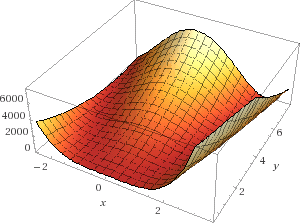The Rosenbrock function for a = 2 and b = 100

First, we will search for which points its gradient $\nabla f(x,y)$ equals zero.

So we compute the partial derivatives and we find:

(Tip: if you want to check your calculation you can use Wolfram alpha)

Our goal is to find when they are both at zero, so we need to solve the following system of equations:

$\begin{eqnarray} 2(200x^3-200xy+x-2) &=&0 \\ 200(y-x^2) &=&0\end{eqnarray}$

We distribute to get:

$\begin{eqnarray}400x^3-400xy+2x-4&=&0 \\200y-200x^2 &=&0\end{eqnarray}$

We multiply $(2)$ by $2x$ to obtain:

We now add $(3)$ and $(5)$ to get:

which simplifies into:

We substitute $x$ in $(4)$

It looks like we have found the point $(x,y) = (2,4)$ for which $\nabla f(x,y)=0$ . But is this a minimum?

The Hessian matrix is :

Let us now compute the Hessian for $(x,y) = (2,4)$

The matrix is symetric, we can check its leading principal minors:

Minors of rang 1:

If we remove the last line and last column the minor $M_{11}$  is $3202$.

Minor of rang 2:

This is the determinant of the Hessian:

$3202\times200-(-800)\times(-800)=400$

All the leading principal minors of the Hessian are positives. It means that the Hessian is positive definite.

The two conditions we needed are met, and we can say that the point $(2,4)$ is a local minimum.

### LOCAL minimum?

A point is called a local minimum when it is the smallest value within a range. More formally:

Given a function $f$ defined on a domain $X$, a point $x^*$ is said to be a local minimum if there exists some $\epsilon > 0$ such that $f(x^*) \leq f(x)\;\text{for all}\;x\;\text{in}\;X$  within distance $\epsilon\$ of $x^*$. This is illustrated in the figure below: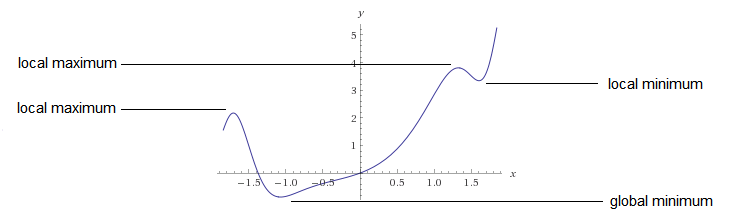A global minimum, however, is true for the whole domain of the function:

Given a function $f$ defined on a domain $X$, a point $x^*$ is said to be a global minimum if $f(x^*) \leq f(x)\quad \text{for all}\;x\;\text{in}\;X$

So all our hard work was just to find a local minimum, but in real life, we often want to find the global minimum...

### How can we find a global minimum?

There is one simple way to find the global minimum:

1. Find all the local minima
2. Take the smallest one; it is the global minimum.

Another approach is to study the function we are trying to minimize. If this function is convex, then we are sure its local minimum is a global minimum.

## Conclusion

We discovered that finding the minimum of a function is not so simple, and it was not even a global minimum. However, some functions, called convex functions are easier to work with. What is a convex function? Read the Part 5 of this tutorial series to find out! Thanks for reading.

# SVM - Understanding the math - the optimal hyperplane

This is the Part 3 of my series of tutorials about the math behind Support Vector Machine.

If you did not read the previous articles, you might want to start the serie at the beginning by reading this article: an overview of Support Vector Machine.

The main focus of this article is to show you the reasoning allowing us to select the optimal hyperplane.

Here is a quick summary of what we will see:

• How can we find the optimal hyperplane ?
• How do we calculate the distance between two hyperplanes ?
• What is the SVM optimization problem ?

## How to find the optimal hyperplane ?

At the end of Part 2 we computed the distance  $\|p\|$ between a point  $A$ and a hyperplane. We then computed the margin which was equal to $2 \|p\|$.

However, even if it did quite a good job at separating the data it was not the optimal hyperplane.Figure 1: The margin we calculated in Part 2 is shown as M1

# SVM - Understanding the math - Part 2This is Part 2 of my series of tutorial about the math behind Support Vector Machines.
If you did not read the previous article, you might want to start the serie at the beginning by reading this article: an overview of Support Vector Machine.

In the first part, we saw what is the aim of the SVM. Its goal is to find the hyperplane which maximizes the margin.

But how do we calculate this margin?

## SVM = Support VECTOR Machine

In Support Vector Machine, there is the word vector.
That means it is important to understand vector well and how to use them.

Here a short sum-up of what we will see today:

• What is a vector?
• its norm
• its direction
• How to add and subtract vectors ?
• What is the dot product ?
• How to project a vector onto another ?

Once we have all these tools in our toolbox, we will then see:

• What is the equation of the hyperplane?
• How to compute the margin?

# SVM - Understanding the math - Part 1 - The margin

## Introduction

This is the first article from a series of articles I will be writing about the math behind SVM. There is a lot to talk about and a lot of mathematical backgrounds is often necessary. However, I will try to keep a slow pace and to give in-depth explanations, so that everything is crystal clear, even for beginners.

If you are new and wish to know a little bit more about SVMs before diving into the math, you can read the article: an overview of Support Vector Machine.

## What is the goal of the Support Vector Machine (SVM)?

The goal of a support vector machine is to find  the optimal separating hyperplane which maximizes the margin of the training data.

The first thing we can see from this definition, is that a SVM needs training data. Which means it is a supervised learning algorithm.

It is also important to know that SVM is a classification algorithm. Which means we will use it to predict if something belongs to a particular class.

For instance, we can have the training data below:

We have plotted the size and weight of several people, and there is also a way to distinguish between men and women.

With such data, using a SVM will allow us to answer the following question:

Given a particular data point (weight and size), is the person a man or a woman ?

For instance:  if someone measures 175 cm and weights 80 kg, is it a man of a woman?